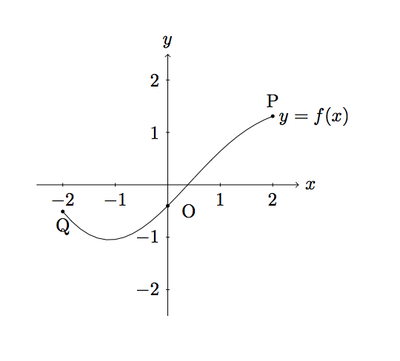# Transforming the graph of a function

Alignments to Content Standards: F-BF.B.3

The figure shows the graph of a function $f$ whose domain is the interval $-2 \leq x \leq 2$.1. In (i)–(iii), sketch the graph of the given function and compare with the graph of $f$. Explain what you see.
1. $g(x) = f(x) + 2$
2. $h(x) = -f(x)$
3. $p(x) = f(x+2)$
2. The points labelled $Q,O,P$ on the graph of $f$ have coordinates
$$Q = (-2,-0.509), \quad O = (0,-0.4), \quad P = (2,1.309).$$ What are the coordinates of the points corresponding to $P,O,Q$ on the graphs of $g,h,$ and $p$?

## IM Commentary

Like "Building functions: concrete case'' this task examines, in a graphical setting, the impact of adding a scalar, multiplying by a scalar, and making a linear substitution of variables on the graph of a function $f$. The setting here is abstract as there is no formula for the function $f$. The focus is therefore on understanding the geometric impact of these three operations. The solution is identical to "Building functions: concrete case'' but the cognitive demand is different because there is no formula to use. This task is appropriate either for assessment or for instruction. It can also be done in combination with "Building a specific quadratic function'' and "Building a general quadratic function'' which develop the idea of "completing the square'' and deriving the quadratic formula.

## Solution

The graphs of $f,g,h,$ and $p$ are shown (within the range of $-2 \leq x \leq 2$) below together to reveal their geometric relationship:1. Looking at the two points $(x,f(x))$ and $(x,g(x))$ we have $$(x,g(x)) = (x,f(x)+2)$$ for every value of $x$. So for each point $(x,f(x))$ on the graph of $f$, there is a corresponding point $(x,f(x)+2)$ on the graph of $g$, located two units above $(x,f(x))$. This means that the graph of $g$ is the same as the graph of $f$, displaced $2$ units upward.
2. The point $(x,h(x))$ is the same as $(x,-f(x))$ so the graph of $h$ is the same as the reflection of the graph of $f$ about the $x$-axis.
3. The point $(x-2,p(x-2))$ is on the graph of $p$. We have $p(x-2) = f(x-2+2) = f(x)$ so the point $(x-2,f(x))$ is on the graph of $p$. Since $(x,f(x))$ is on the graph of $f$ and $(x-2,f(x))$ is $(x,f(x))$ shifted two units to the left, we conclude that the graph of $p$ is the graph of $f$ translated two units to the left.

1. The graph of $g$ lies $2$ units above the graph of $f$. So, denoting by $Q_g,O_g,P_g$ the points on the graph of $g$ corresponding to $Q,O,$ and $P$ on the graph of $f$ we have $Q_g = (-2,1.491)$, $O_g = (0,1.6)$, and $P_g = (2,3.309 )$. For $Q_h,O_h,$ and $P_h$ the relationship of $h$ to $f$ is that its output has the opposite sign. So we will find $Q_h = (-2,0.509)$, $O_h = (0,0.4)$, and $P_h = (2,-1.309)$. Finally for $Q_p, O_p,$ and $P_p$, the relationship of $p$ to $f$ is that it shifts the graph of $f$ to the left by two units, so $Q_p = (-4,-0.509)$, $O_p = (-2,-0.4)$, and $P_p = (0,1.309)$.